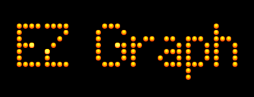# Conic Sections - The Parabola

On an (x, y) graph a parabola can have the shape to the left.Here's a link to EZ Graph - Parabola. This is a graphic calculator that presents functions that draw parabolas.

If a plane intersects the cone when it is slanted the same as the side of the cone, (formally, if a plane is parallel to the cone's slant height), then the conic section is a parabola. This is shown to the left.This link will take you to an interactive 3D virtual reality world showing the parabola as a conic section.

Here is a video that shows several possibilities for forming a parabola by intersecting a cone and a plane.

Custom Search